## research papersFOUNDATIONSADVANCES
ISSN: 2053-2733
Volume 73| Part 2| March 2017| Pages 93-101

## Asymmetry in serial femtosecond crystallography data

aDepartment of Chemistry and Molecular Biology, University of Gothenburg, Box 462, Gothenburg 40530, Sweden, and bDepartment of Chemistry, Bridge Institute, University of Southern California, Los Angeles, CA 90089, USA
*Correspondence e-mail: gergely.katona@cmb.gu.se

(Received 7 July 2016; accepted 22 November 2016; online 30 January 2017)

Serial crystallography is an increasingly important approach to protein crystallography that exploits both X-ray free-electron laser (XFEL) and synchrotron radiation. Serial crystallography recovers complete X-ray diffraction data by processing and merging diffraction images from thousands of randomly oriented non-uniform microcrystals, of which all observations are partial Bragg reflections. Random fluctuations in the XFEL pulse energy spectrum, variations in the size and shape of microcrystals, integrating over millions of weak partial observations and instabilities in the XFEL beam position lead to new types of experimental errors. The quality of Bragg intensity estimates deriving from serial crystallography is therefore contingent upon assumptions made while modeling these data. Here it is observed that serial femtosecond crystallography (SFX) Bragg reflections do not follow a unimodal Gaussian distribution and it is recommended that an idealized assumption of single Gaussian peak profiles be relaxed to incorporate apparent asymmetries when processing SFX data. The phenomenon is illustrated by re-analyzing data collected from microcrystals of the Blastochloris viridis photosynthetic reaction center and comparing these intensity observations with conventional synchrotron data. The results show that skewness in the SFX observations captures the essence of the Wilson plot and an empirical treatment is suggested that can help to separate the diffraction Bragg intensity from the background.

### 1. Introduction

X-ray free-electron lasers (XFELs) are linear accelerator based X-ray sources that deliver a peak X-ray brilliance a billion times greater than synchrotron radiation (Emma et al., 2010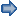). These revolutionary machines were foreseen to create new possibilities in life science (Neutze et al., 2000) and over the six years since XFELs have been available to users the rapid pace of development has been impressive (Schlichting, 2015). One major application of XFEL radiation is the development of serial femtosecond crystallography (SFX). A proof-of-principle study first performed at low resolution using crystals of photosystem I (Chapman et al., 2011) was shortly afterwards extended to high resolution (Boutet et al., 2012) and has since been applied to time-resolved X-ray diffraction (Tenboer et al., 2014), the study of protein–protein receptor complexes (Kang et al., 2015) and de novo phasing (Barends et al., 2014). Serial crystallography has also since been applied to studies using synchrotron radiation (Nogly et al., 2015) and is expected to become a broadly applied method at storage ring based microfocus beamlines.

Classical synchrotron-based protein crystallography records diffraction images by rotating a protein crystal within an X-ray beam and thereby samples data from all unique reflection planes. In contrast, SFX is a random sampling method whereby complete X-ray data are obtained by processing diffraction images collected from thousands of randomly oriented microcrystals as they are continuously streamed across the path of a highly intense XFEL beam (Chapman et al., 2006). This strategy creates new challenges and the last few years have seen the emergence of various experimental and data processing tools designed to handle such nuances. CrystFEL (White et al., 2012) is an SFX data analysis software suite which performs indexing by calling DirAx (Duisenberg, 1992), XDS (Kabsch, 2010) and MOSFLM (Leslie, 2006). CrystFEL then models diffraction spots as circular regions surrounded by an annulus masking background pixels, similar to early versions of Denzo (Otwinowski & Minor, 1997). Diffraction intensities are quantified by integrating over all pixels occupied within the selected regions, and the spot intensity is calculated either by subtracting the total peak counts from that estimated from the background region, or by using profile fitting (Rietveld, 1969).

A Monte Carlo merging approach in CrystFEL calculates merged intensity for the unique reflections by taking a simple mean of all the symmetrically equivalent observations (Kirian et al., 2010). This is appropriate when the corresponding intensities follow a unimodal normal distribution. An alternative to CrystFEL which is based upon an extrapolation method assumes that all the crystals in an SFX experiment are identical and the final energy distribution in the reciprocal space is Gaussian (Zhang et al., 2014). Microcrystals possess very different diffraction power and the detector distance cannot be optimized for each crystal as in a conventional synchrotron-based experiment. The data collection parameters are instead optimized to capture the highest-resolution reflections from the best ordered crystals. This also means that many high-resolution reflections without signal are integrated from the large body of weakly diffracting crystals. Although attempts are made to limit the integration radius to that of the crystal resolution, this point estimate is error prone. As a result, intensity observations may not necessarily follow a single normal distribution.

Here, we explore an alternative to the perfect Gaussian distribution for serial crystallography data using data recorded at the LCLS (Linac Coherent Light Source, Stanford University, California) from microcrystals of the Blastochloris viridis (B. viridis) photosynthetic reaction center (RCvir) as a representative example. We examine the asymmetry in the reflection intensities using an ex-Gaussian distribution as a minimal extension of a Gaussian distribution and contrast the asymmetry in SFX data to more conventional X-ray diffraction observations from a single crystal collected at a synchrotron source. Finally, we make a preliminary proposal for how the properties of an ex-Gaussian distribution may be exploited to infer the Bragg intensities of an idealized crystal' in the microcrystal slurry.

### 2. Materials and methods

#### 2.1. Growth and purification of reaction center from B. viridis

The photosynthetic reaction center from B. viridis was cultivated and purified as described by Johansson et al. (2013).

#### 2.2. Lipidic sponge phase batch crystallization

Lipidic sponge phase was prepared as described by Wöhri et al. (2009) with the following modifications. Melted monoolein was thoroughly mixed in a ratio of 3:2 (v/v) with 0.1 M HEPES pH 7.5, 0.1% LDAO until a viscous transparent lipidic cubic phase was obtained. The formed phase was then transferred into a glass vial and sponge-phase-inducing solution (1:4 ratio) was added containing 16% Jeffamine M-600, 1 M HEPES pH 7.9, 0.7 M ammonium sulfate, 2.5% 1,2,3-heptanetriol, which swells the cubic phase to the sponge phase. After phase separation overnight, the upper phase (sponge phase) was harvested. Crystals were grown using batch crystallization in the lipidic sponge phase. Equal amounts of sponge phase and protein were mixed in a 4:1 ratio (v/v) with 1.2 M tris-sodium citrate (2 parts sponge phase, 2 parts protein, 1 part tris-sodium citrate) and allowed to incubate for several weeks. Crystals grew in the dark at room temperature and were delivered to the CXI (coherent X-ray imaging) instrument using a sample loop. The protein concentration used for crystallization setups was 20 mg ml−1 with an optical purity ratio of A280/A830 = 2.27.

#### 2.3. Synchrotron single-crystal data collection

Single-crystal X-ray data were collected under cryo-conditions with a 225 mm MarMOSAIC CCD detector at ID23-2 at the ESRF (Grenoble, France) (λ = 0.8726 Å). The oscillation range per image was 1°, the exposure time 1.0 s, the distance to the detector 373.1 mm. The crystals belong to the space group P212121 with unit-cell dimensions of a = 57.6, b = 82.9, c = 382.1 Å, α = β = γ = 90°.

#### 2.4. SFX data processing and analysis

RCvir_XFEL data from 1175 images were indexed and integrated using the indexamajig program of the CrystFEL 0.6.2 software suite. Data were indexed using the space group P212121 with unit-cell dimensions of a = 57.9, b = 84.8, c = 384.3 Å, α = β = γ = 90° and data reflections were integrated up to the apparent diffraction limit of each crystal by using the _rescut _push-res = 0 option. Three parallel data processing approaches, namely CrystFEL process_hkl, CrystFEL partial­ator and the Ideal Crystal approach, were used to merge data. The CrystFEL process_hkl scaled and merged the data using the Monte Carlo method; the CrystFEL partialator method was used to merge the data using the partiality scgaussian' model with data scaling using three iterations. Thirdly, the Ideal Crystal approach which is based on the Markov chain Monte Carlo (MCMC) method (Gilks et al., 1996) was implemented using the pymc3 library (Salvatier et al., 2016) and the unmerged data which were sorted and arranged in the group of unique reflections using the cctbx libraries (Sauter et al., 2013). The data collection and processing statistics using all three approaches are summarized in Table 1. Wilson B factors for the Ideal Crystal approach were matched with those of CrystFEL process_hkl and Cryst­FEL partialator approaches by multiplying the intensities and σ's by a correction factor [f = b − (b − 1)q2/q2n, where b is a variable, q is the inverse resolution squared (1/d2) and qn is the q of the highest-resolution reflection]. Two sets of the Ideal Crystal data were generated using correction factors with b = 1.05 and b = 1.19 in order to compare with the CrystFEL process_hkl and partial­ator approach, respectively. All four data sets were processed using CCP4 7.0.020 (Winn et al., 2011) truncate software (French & Wilson, 1978).

 Table 1Data collection, processing and refinement statistics
 M = Multiplicity for the reflection (h, k, l). LLG = final log-likelihood gain after molecular replacement (MR) by Phaser.
Crystal RCvir_XFEL CrystFEL RCvir_XFEL Ideal Crystal RCvir_Sync
Resolution (Å) 55.44–3.5 (3.6–3.5) 55.44–3.5 (3.6–3.5) 50.52–3.6 (3.8–3.6)
a, b, c (Å) 57.9, 84.8, 384.3 57.9, 84.8, 384.3 57.6, 82.9, 382.1
α, β, γ (°) 90, 90, 90 90, 90, 90 90, 90, 90
Space group P212121 P212121 P212121
Completeness (%) 99.9 (99.8) 99.5 (99.3) 98.9 (92.7)
Unique reflections 24941 (1991) 24932 (1977) 22398 (2986)
Multiplicity 59 59 7
Data processing method Process_hkl Partialator Correction factor with b = 1.05 Correction factor with b = 1.19 XDS
I/σ 3.5 (2.1) 3.9 (2.1) 6.1 (4.8) 4.1 (1.2)
Wilson B factor (Å2) 26.6 59.6 26.6 59.6 37.6
LLG 3489.50 1565.66 3963.70 4410.41 3333.70
Rfree/Rwork (%) 27.4/25.3 31.2/28.6 27.3/25.3 26.6/24.7 28.0/24.2
r.m.s.d. bonds (Å) 0.003 0.004 0.003 0.003 0.016
r.m.s.d. angles (°) 0.712 0.744 0.701 0.725 1.57

For structural comparison between the Ideal Crystal data sets and those from CrystFEL data processing approaches, all four diffraction data sets were cut at 3.5 Å resolution. Mol­ecular replacement (MR) solutions were searched in the P212121 space group with a search model of RCvir (PDB entry 4cas, Johansson et al., 2013) using Phaser-MR in Phenix version 1.10-2155-1692 (Adams et al., 2010). The MR solutions using Ideal Crystal data sets showed the higher log-likelihood gains among the tested data processing strategies (shown in Table 1). RCvir_XFEL structures were refined for all four data sets using the same refinement strategies in the Phenix suite. The refinement strategy implemented was performed using five cycles of refinement for coordinates, real space, rigid body and individual B factors. The refinement protocol was further modified by using maximum likelihood target functions and the best weights for the X-ray target functions, and the B-factor restraints were optimized. Water update and automatic correction of N/Q/H errors were kept active during the refinement. Refinement statistics are summarized in Table 1which shows that refinement parameters are quite similar between the corresponding Ideal Crystal and CrystFEL approaches. Simulated annealing composite omit maps were calculated in Phenix for all four data sets. All the maps were contoured at 1σ.

### 3. Results

#### 3.1. Overview of data processing steps

The goal of any crystallographic analysis of diffraction patterns is to present the data in a way that the structural refinement packages can use. The new concept of serial crystallography (Chapman et al., 2011) deals with very high multiplicity, but the underlying principles are the same as in traditional approaches to crystallography in that serial crystallography records multiple observations for any unique reflection from multiple images, and then merges these observations to yield Ihkl and σhkl which scaling and other crystallographic programs can use. The data processing scheme used here starts with the indexing and integration of the diffraction images. Complete diffraction data are then obtained in the form of a stream file containing the list of all the observations. These observations are then mapped to the asymmetric unit using the cctbx Python libraries (Grosse-Kunstleve et al., 2002). The key scientific idea here is to determine the distribution of the observations, and the histogram for each reflection is calculated and fitted with different distribution functions. The fitting parameters are then used to diagnose and determine the Bragg reflection and diffuse intensity response.

#### 3.2. Symmetric and asymmetric distribution functions

Intensity distributions for all the unique reflections were calculated and fitted using selected distribution functions. Standard approaches of processing crystallographic data assume an idealized Gaussian distribution function:where μ and σ provide information about the mean and variability in the reflection, respectively. Serial crystallography risks merging many more weak observations than expected when using classical data collection strategies and therefore the distribution of observations may be expected to be skewed towards a larger number of weak observations. To test this possibility, we also consider a minimal extension of the Gaussian distribution known as an ex-Gaussian distribution function which is a convoluted distribution of a Gaussian and an exponential distribution function and is represented as:where erf is an error function with erf(0) = 0; erf(∞) = 1. Here, μ and σ provide information on localization and variability of the Gaussian part, respectively, and τ is the mean of an exponential component, providing information about the degree of skewness in the distribution of reflection intensities.

A parameter search algorithm using maximum likelihood estimation (Lacouture & Cousineau, 2008) was used to recover optimal parameters for both Gaussian and ex-Gaussian distributions to crystallographic observations for any given hkl Miller indices. For the parameter value (p) which allows a correct fit of the distribution function f(x|p) to the data (x), the minus log-likelihood criterion was applied in which the minimum of a −LogL is estimated, where LogL for a parameter value p is defined asScanning through all possible values of the parameter is time consuming, specifically when fitting with multi-parameter distribution functions. Therefore, as a first approximation the local minimum of the function was reached by iteratively adjusting the starting parameter values using the Simplex method based on the steepest gradient algorithm (Lacouture & Cousineau, 2008). We later extend the inference within the a posteriori parameter space using the MCMC algorithm (Gilks et al., 1996) to estimate the uncertainty in distribution parameters.

#### 3.3. Expectation value calculation using XFEL data

X-ray diffraction images were collected by injecting thousands of microcrystals of RCvir into the XFEL pulses coming with a repetition rate of 120 Hz at the LCLS. Images were indexed and integrated using the indexamajig program of the CrystFEL suite (White et al., 2012). All the indexed observations for each microcrystal are collectively put together into a complete diffraction data set in one stream file. Each batch contains a set of partial reflections which were re-assembled to have all the equivalent reflections in an order. All the equivalent observations were then mapped to the asymmetric unit of space group P212121 using the cctbx Python library through the map_to_asu function of Miller array objects.

XFEL diffraction RCvir data (RCvir_XFEL) were considered up to 3.5 Å resolution as previously described (Johansson et al., 2013). We calculated the histogram for the distribution of observations for each reflection and fitted it with both a Gaussian and an ex-Gaussian distribution profile. Figs. 1(a)–1(d) show the Gaussian (red) and the ex-Gaussian (blue) fits to the histograms for four reflections selected at different resolutions. The ex-Gaussian fit was observed to provide a better shape fit to the histogram than the Gaussian fit. Table 2provides the values of the fitting parameters obtained using the two distributions for the selected reflections. μg and σg are the fitting parameters obtained using the Gaussian fit whereas μexg, σexg and τexg are the ones obtained using the ex-Gaussian fit. Consistent with the definition of an ex-Gaussian distribution, we observed that for the reflections which have relatively reduced skewness the ex-Gaussian approaches a normal distribution, as is seen for the reflection (1, 21, 54) in Fig. 1(d). In contrast, for the reflection (7, 4, 5) in Fig. 1(b), where skewness is high, the ex-Gaussian distribution approaches an exponential distribution.

 Table 2Fitting parameters using a Gaussian and ex-Gaussian distribution for a selected set of reflections from the RCvir data collected at the XFEL
 M = multiplicity for the reflection (h, k, l). χ2 = chi-square value calculated by taking twice the difference between the negative of the log-likelihood of a Gaussian and an ex-Gaussian for a single degree of freedom.
h k l Resolution (Å) M χ2 μg σg μexg σexg τexg
1 21 54 3.50 57 82.6 1022.0 253.6 −532.0 543.3 1594.1
0 22 0 3.85 23 1.1 886.9 281.9 −109.6 1096.5 1036.4
7 17 9 4.25 77 198.5 2355.6 519.0 −763.4 597.2 3172.2
0 0 90 4.25 5 10.7 1491.9 624.9 −228.1 1234.8 2365.5
7 4 5 7.67 119 449.2 7496.7 1330.3 −568.4 621.2 8152.9
0 0 50 7.65 6 0 1.5 211.4 −597.5 511.3 711.5
2 0 5 27.1 158 480.4 7707.6 1044.5 −1070.6 637.1 8871.5
0 0 14 27.3 14 24.1 1331.6 720.7 −1386.7 599.7 2957.4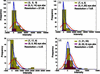Figure 1 (a)–(d) Histograms for the distribution of some of the unique Bragg reflections (yellow) and the systematically absent reflections (magenta) selected from the resolution shells around 27.3, 7.6, 4.2 and 3.8 Å, respectively, from the RCvir_XFEL data. The fits to these distributions using a Gaussian (red) and an ex-Gaussian (blue) function are shown. The fits to the Bragg reflections and the systematically absent reflections are shown as full and dashed lines, respectively. Miller indices for the reflections used are given in parentheses.

A Gaussian is a subset of an ex-Gaussian function; hence a fit with an ex-Gaussian will always be better than or equal to that of a Gaussian. In order to check the desirability of such a distribution function to correctly model the frequency of intensity observations, we performed a likelihood ratio test for nested functions. In this test, twice the difference between the negative of the log-likelihood of the Gaussian and ex-Gaussian provides the chi-square estimate for an extra degree of freedom. Chi-square values for each reflection were calculated and the decision to fit the reflection using an ex-Gaussian distribution profile was taken on the basis of 5% chance criteria, which gives a chi-square cutoff score of 3.84 for a single degree of freedom. For RCvir_XFEL data, it was found that 95% of reflections strongly favour an estimation to be made using an ex-Gaussian distribution function. This preference for an ex-Gaussian over a Gaussian fit was found to be consistent over the complete resolution range (Fig. 2a).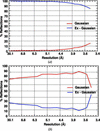Figure 2 Percentage of reflections from (a) RCvir_XFEL and (b) RCvir_Sync data that could be best explained using a Gaussian (red) and an ex-Gaussian (blue) distribution.

To explore further the information contained in a Gaussian and the exponential parts of an ex-Gaussian distribution, we plotted the mean (μexg) and the skewness (τexg) parameters of the ex-Gaussian function as a function of resolution (Fig. 3a). At high resolution (above 7 Å), τexg follows the Wilson distribution in a similar manner to μg of the Gaussian function. τexg and μg have the same magnitude in the high-resolution range and show standard maxima and minima at 4.5 Å and 6 Å, respectively. On the other hand, μexg does not appear to follow the expected Wilson intensity distribution at high resolution.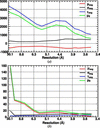Figure 3 This shows the variation in the mean (μexg, red) of the Gaussian part of an ex-Gaussian fit, the standard deviation (σexg, black), the skewness mean (τexg, blue) from the exponential part of the ex-Gaussian fit and the mean intensity of the reflection (μg, green) with the resolution for (a) RCvir_XFEL and (b) RCvir_Sync data.

#### 3.4. Comparison with the systematic absences

The magenta and yellow coloured histograms in Figs. 1(a)–1(d) show the distributions of the four systematically absent and non-absent Bragg reflections, respectively, in different resolution ranges, 27.3, 7.6, 4.2 and 3.8 Å. Comparing the histograms of the Bragg reflections at high resolutions with those of the systematically absent reflections in our data, we observe that the most frequent intensity observations of non-absent Bragg reflections overlap with those of the systematically absent reflections present in the same resolution range. In addition, there is a small fraction of valuable observations that are responsible for the extended tail of the distribution. Curiously, not even at the lowest-resolution reflection (27.3 Å in Fig. 1a) is the distribution symmetric and we observe that there is no genuine shift in the most frequent observations compared with the systematic absence at similar resolution.

#### 3.5. Comparison with synchrotron data

A similar analysis of reflection intensities was carried out using diffraction data collected at a synchrotron source (ESRF) from a single macrocrystal of RCvir. Lipidic sponge phase microcrystals had a different space group from the earlier form of the macrocrystals (Johansson et al., 2012). To make a fair comparison, we have reproduced an RCvir macrocrystal to match the crystal packing with that of the microcrystals used for XFEL-based data collection. Although we were able to produce macrocrystals in the P212121 space group, they diffracted only up to 3.6 Å in contrast to the 1.86 Å limit achieved earlier by using the RCvir crystals produced in the P21212 space group (Wöhri et al., 2009). Table 1provides the crystallographic data statistics showing the comparison between the data collected at the synchrotron source to 3.6 Å and those collected at the XFEL source at 3.5 Å. The synchrotron data (RCvir_Sync) have smaller multiplicity in comparison with the XFEL data.

It is often sufficient that crystals are indexed with the help of their low-resolution reflections, but the measured intensities at predicted spot positions can be practically zero at higher resolution in the case of low-quality crystals. Fig. 4(a) shows an SFX diffraction snapshot from a weakly diffracting crystal where high-resolution reflections are not observed; nevertheless Bragg positions are integrated beyond the visible limit of Bragg spots. In synchrotron crystallographic data collection (Fig. 4b), a similar large variation in diffraction intensity is not observed unless the diffraction is very anisotropic or the crystal is poorly centered. For this reason, we see that the histogram of synchrotron reflections is more symmetric and can be modeled unambiguously with a Gaussian distribution (Fig. 2b). If fitted using an ex-Gaussian distribution, the tail is less pronounced and τexg has little influence on the mean intensity of the reflection (Fig. 3b). Conversely, in SFX data only a few good-quality crystals produce overall high-intensity observations leading to a tail in the intensity histogram.Figure 4 Diffraction quality of RCvir_XFEL and RCvir_Sync. A representative diffraction image collected from (a) microcrystals of RCvir at the CXI (coherent X-ray imaging) beamline of the LCLS and (b) a crystal of RCvir on the ID23-2 beamline of the ESRF.

#### 3.6. Empirical inference of Bragg intensity from idealized crystals

When developing a statistical method, it is important to define the goal of the analysis. The presumed goal of current practice is to describe the central tendency of the data and the variability of observations. It is expected that partiality correction and image-by-image scaling reduce the variability of observations, but this does not change the original goal. If the distribution is symmetric and unimodal the statistical mean could estimate the most frequent intensity observation and our data show that this is a valid assumption for most of the Bragg intensities recorded from a single crystal of reaction center at a synchrotron. There are two serious problems with the original goals when it comes to SFX data. Firstly, we have shown that the distribution is very asymmetric, the mean is ill suited to describe the central tendency of the distribution and the standard errors determined from such distributions cannot accurately describe the confidence interval of the mean. Secondly, the comparison of systematic absences and non-absent Bragg reflections at similar resolution shows that their mode is essentially the same; therefore, it is potentially misleading to try to determine (more accurately) the most frequent intensity of non-absent Bragg reflections. On the other hand, we are free to choose more meaningful goals for the statistical analysis, for example by replacing the question what is the typical observed Bragg reflection intensity in an experiment?' with how high can the Bragg intensity be in an experiment?'. While the typical intensity observation is strongly influenced by diffuse scattering, the highest ones are more likely to originate from good crystals. Thus, we can aim to determine the diffraction intensity of an idealized' crystal in its ideal diffraction condition. The highest-intensity observation of any given unique reflection may or may not originate from this group of ideal crystals at the top of their rocking curve, but we can define a region of the intensity probability distribution where we expect the ideal crystal reflections to appear even in the absence of an actual observation. This depends on how restrictively the ideal region is defined and the multiplicity of observations.

As a first step, we have developed a Bayesian model to infer the probability distribution of the parameters of the empirical ex-Gaussian distribution from the intensity observations of each Bragg reflection using a minimally informative prior knowledge (assuming a uniform distribution). We have approximated the posterior distribution (given the intensity observations) using the MCMC method (Gilks et al., 1996) based on a similar probabilistic model that was previously used for determining the structure amplitude difference of correlated intensity observations (Katona et al., 2016). For each Bragg reflection we determined the maximum a posteriori values of model parameters (μexg, σexg, τexg) as a starting point and calculated 50 000 Monte Carlo samples [Metropolis stepping (Metropolis et al., 1953)], the first 40 000 of which we discarded. We performed thinning of the Markov chain by taking every tenth sample from the posterior joint probability distribution and determined the intensity value (Iideal) at which the cumulative distribution function (c.d.f.) of each ex-Gaussian distribution sample reached a critical value (0.95). The optimal level of critical cumulative probability can be set empirically, but ideally it should not be less than the expected frequency of the diffuse scattering intensity response. A representative example illustrating the posterior distribution samples and corresponding Iideal positions is shown in Fig. 5. Finally, we have defined the intensity of the idealized crystals as the mean of Iideal and its uncertainty as the standard deviation of Iideal.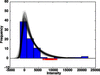Figure 5 Ex-Gaussian distribution profiles using the Markov chain Monte Carlo a posteriori estimates (plots in black) for a Bragg reflection whose intensity histogram is shown in blue. Shown in red is the spread of the intensity value (Iideal) at which the c.d.f. of ex-Gaussian distributions reaches 0.95.

These idealized data were further processed with truncate of the CCP4 package in parallel with the CrystFEL process_hkl and partialator data as reference data sets. The Ideal Crystal approach resulted in a flatter Wilson plot than the data processed by CrystFEL process_hkl (15 Å2 versus 27 Å2, respectively). These Wilson plots are comparable with single-crystal synchrotron data recorded to similar resolution (37.6 Å2) (Fig. 6), but the partiality-corrected data set is substantially steeper even if it originates from the same set of observations. This is not very surprising since many traditional crystallographic properties have weaker theoretical foundations in SFX. In particular, the observed Wilson plot cannot be directly related to the random displacement of atoms in the unit cells of a single crystal since each crystal has different diffractive power, mosaic spread, atomic displacement parameter distribution etc. Thus, the Wilson plot and the derived Wilson B factor lose their traditional physical meaning without further information about the distribution of the microcrystals. This is not necessarily a problem since empirical tools can be employed to approximate the SFX observations to those of single-crystal diffraction or to improve the quality of the electron-density maps or refinement. Partialator scaling tries to incorporate a crystal-specific scaling factor and B factor to compensate for this heterogeneity in SFX data sets, but the question then becomes what is the optimal ultimate scale target?'.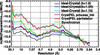Figure 6 Wilson plots for the RCvir_XFEL data generated using Ideal Crystal (b = 1), Ideal Crystal (b = 1.05), Ideal Crystal (b = 1.19), CrystFEL _push-res = 0 process_hkl and CrystFEL partialator _model = scgaussian options are shown in grey, blue, cyan, magenta and red, respectively. Wilson plot for the RCvir_Sync data is shown in green.

We have applied a correction factor [b − (b − 1)q2/q2n, where b is a variable, q is the inverse resolution squared (1/d2) and qn is the q of the highest-resolution reflection] to the intensities and σ's prior to the truncate step to match the Wilson B factor of the Ideal Crystal approach with that of the process_hkl and partialator data (26.6 and 59.6 Å2, respectively, after the correction) to allow a fair comparison. The resulting Wilson plots are shown in Fig. 6. The I/σ (Fig. 7) determined by the Ideal Crystal approach appears to be higher and reflects an appropriate accuracy of the measurement. This is strongly influenced by the choice of the c.d.f. critical value (0.95).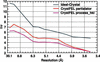Figure 7 Plots showing I/σ versus resolution (Å) for the RCvir_XFEL data indexed using _push-res = 0 option of CrystFEL. Plots in grey, red and magenta correspond to three different data processing methods – Ideal Crystal, CrystFEL partialator _model = scgaussian and CrystFEL process_hkl, respectively.

Subsequent modeling steps and map calculations were performed in the Phenix suite (Adams et al., 2010) using identical protocols (see §2). After molecular replacement by Phaser the final log-likelihood gain was higher when using the Ideal Crystal data (Table 1). The best Rfree value was obtained from the Ideal Crystal approach when scaled to the partialator data, but at the same time the omit-map quality became worse, although not to the same extent as seen in the partialator data. When scaled to the process_hkl data the Rfree was slightly lower using the Ideal Crystal approach than process_hkl (27.3% versus 27.4%), and the resulting figures of merit, which provide a measure of phase quality for each reflection, were higher (Fig. 8).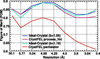Figure 8 Figure of merit (a measure of phase quality) versus resolution plots for the RCvir_XFEL data generated using Ideal Crystal (b = 1.05), CrystFEL process_hkl, Ideal Crystal (b = 1.19) and CrystFEL partialator _model = scgaussian options are shown in blue, magenta, cyan and red, respectively.

Fig. 9presents a comparison of simulated annealing omit maps calculated from the two data sets – Ideal Crystal (b = 1.05) and process_hkl. Map comparison [Ideal Crystal (blue) versus process_hkl (magenta)] for four selected regions of RCvir, i.e. residues 57–67 in cytochrome C subunit, residues 47–50 in the intra-membrane subunit L, residues 65–68 in the intra-membrane subunit L and the mena­quinone in subunit M, is shown. In summary, the process_hkl and Ideal Crystal data sets yield electron-density maps and other quality indicators that are broadly comparable, with the Ideal Crystal data sets in some respects seeming better, without any extensive optimization.Figure 9 Simulated annealing composite omit maps calculated using RCvir_XFEL data sets processed using the Ideal Crystal (b = 1.05) approach (blue) and CrystFEL process_hkl option (magenta). Selected regions of RCvir subunits are shown in four different panels: (a) residues 57–67 in cytochrome C subunit, (b) residues 47–50 in the intra-membrane subunit L, (c) residues 65–68 in the intra-membrane subunit L and (d) the mena­quinone in subunit M. Residues are shown in element colour.

It will ultimately be necessary to distinguish distributions that are likely to contain at least one Bragg intensity observation from those that do not contain any. Although one may expect that the best Bragg reflections are at the high end of the c.d.f., the converse is not necessarily true and some observation distributions are presumed to not contain any measurable Bragg reflections at all. In the absence of a genuine observation a prior estimate has to be used. These efforts may be greatly facilitated by the intensity observations of systematic absences as they can help to define the low end of the dynamic range at each resolution bin. As a further development, it may also be possible to optimize the choice of the critical c.d.f. probability: at a higher value one may expect even better selectivity, at the expense of increased uncertainty of Iideal. One may also find better ways to analyze the posterior distribution of Iideal (reporting the median or mode) and alternative ways to describe their dispersion (for example, using median absolute deviation). Our procedure does not necessitate the use of an ex-Gaussian distribution; in principle, any asymmetric distribution (uni- or multimodal) may be applied. Once the most successful empirical distribution is identified, a suitable outlier recognition method can be developed. Since genuine Bragg intensity observations may be a small fraction of the multiplicity, it may be beneficial to incorporate more a priori information in the Bayesian model (using empirical Bayes methods or from first principles).

### 4. Conclusion

SFX experiments can produce highly heterogeneous data and our primary purpose was raising the awareness of their incorrect treatment. We observed that in the SFX data the skewness of the intensity observations follows the characteristic trend of a protein Wilson plot; the same is not true for the intensity of the most frequent observations. The most frequent intensity observations do not appear to originate from Bragg reflections of protein. To deal with the weak diffraction images one possibility is to pre-filter the reflection observations using a dynamic resolution cutoff per image basis. This approach risks rejecting weak observations that otherwise contain useful information, and indeed we have not observed a sharp distinction between the diffuse scattering signal and Bragg reflections. Instead, the information content can be judged from the distribution of the entire data set and the described ex-Gaussian diagnostics and our intensity inference strategy is a step in this direction.

### Acknowledgements

The authors acknowledge the LCLS, a national user facility operated by Stanford University on behalf of the DOE, for collection of the SFX data. The single-crystal X-ray diffraction experiment was carried out at the ID23-2 beamline of the ESRF. This research was funded by the Swedish Science Research Council (VR) and the Knut and Alice Wallenberg Foundation. Data and the protein coordinates have been deposited with PDB codes 5m7k, 5m7j and 5m7l.

### References

This is an open-access article distributed under the terms of the Creative Commons Attribution (CC-BY) Licence, which permits unrestricted use, distribution, and reproduction in any medium, provided the original authors and source are cited.FOUNDATIONSADVANCES
ISSN: 2053-2733
Volume 73| Part 2| March 2017| Pages 93-101